# Divisor problems

Problems in number theory concerned with the asymptotic behaviour of the summation functions $$D(x) = \sum_{n \le x} \tau(n)\ ,\ \ D_k(x) = \sum_{n \le x} \tau_k(n)$$ where $\tau(n)$ is the number of divisors of $n$ and $\tau_k(n)$, $k \ge 2$, is the number of representations of $n$ as the product of $k$ natural numbers, as well as modifications of these functions.

## Dirichlet's divisor problem.

This is the problem of the best estimate of the remainder term $\Delta(x)$ in the asymptotic formula $$\sum_{n \le x} \tau(n) = x \log x + (2 \gamma - 1)x + \Delta(x)$$ where $\gamma$ is the Euler constant. The asymptotics of the sum $$\sum_{n \le x} \tau(n) = D(x)$$ was first considered by P. Dirichlet in 1849. He based himself on the fact that this sum is equal to the number of points $(u,v)$ with positive integer coordinates under the hyperbola $uv = x$, and showed that $$D(x) = x \log x + (2 \gamma - 1)x + O(\sqrt x)$$ This formula is known as Dirichlet's formula for the number of divisors.

The divisor problem was one of the models on which methods for estimating the number of integer points in expanding domains of various types were developed. Let $\theta$ be the greatest lower bound of the numbers $\alpha$ in the relation $\Delta(x) \ll x^\alpha$. According to Dirichlet, $\theta \le 1/2$. G.F. Voronoi proved that $\theta \le 1/3$. Later, the following estimates were obtained in succession: $$\theta \le \frac{33}{100}\ ,\ \ \ \theta \le \frac{27}{82}\ ,\ \ \ \theta \le \frac{15}{46}\ ,\ \ \ \theta \le \frac{13}{40}\ .$$ The true order of the value of $\theta$ is unknown (1988). According to one hypothesis, $$\Delta(x) \ll x^{1/4} \log^2 x \ .$$ On the other hand, it was proved by G.H. Hardy that $\theta \ge 1/4$ or, more exactly, that $$\liminf \frac{\Delta(x)}{(x\log x)^{1/4}\log\log x} < 0 < \limsup \frac{\Delta(x)}{(x\log x)^{1/4}\log\log x}$$

In addition another formula, $$\int_0^x \Delta(y)^2 dy = A x^{3/2} + O(x \log^5 x)$$ where $A$ is a constant, is known; this formula proves that the hypothesis on the order of $\Delta(x)$ is valid "on the average" .

## The generalized divisor problem.

This is the problem of the best asymptotic expression for the sum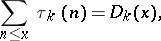as; in particular, if,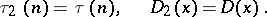The generalized divisor problem is closely connected with the behaviour of the Riemann zeta-function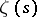in the critical strip of values of. In fact, the following formula is valid for non-integer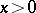,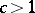:Here, the integrand has at the point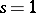a pole of orderwith residue of the formwhereis a polynomial of degree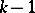.

Let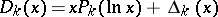and let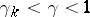, whereis the greatest lower bound of the numbersfor whichThe following formulas are then valid:and the inverse Mellin formula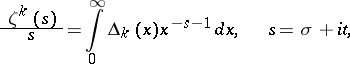where the integral exists in the mean-square sense for.

Estimates of the rest termin the formula for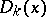are still (1988) far from what might be expected. Let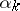be the smallest of the numbersfor which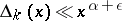for any. The following estimates are known: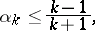These estimates can be rendered more precise for individual values of:The last result giving an estimate from above forwas obtained in  by developing the idea of the Vinogradov method: There is an absolute constant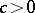such that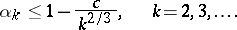This estimate follows from an estimate ofin the critical strip: For,there is a constant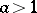such thatOn the other hand, it was shown by Hardy that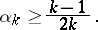Regarding the value of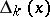, there is the hypothesis thatfor all. However, it cannot be justified, even not by solving the Lindelöf hypothesis:for arbitrary,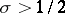.

A further generalization of divisor problems is as follows : Uniformly with respect to the integers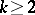,, for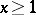one haswhere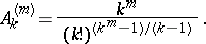## The divisor problem in arithmetical progressions.

This is the problem of estimating the sums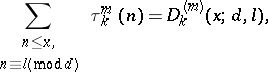uniformly with respect to,,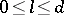,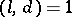. These sums were studied by analytical methods of the theory of-functions, and are important to numerous problems in the theory of numbers . In the simplest case () asymptotic expressions have been obtained:

if, for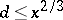(cf. );

if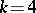, for(cf. );

if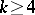, for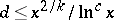(cf. ). For any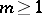and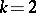, the true order of growth (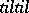) has been found  for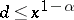,: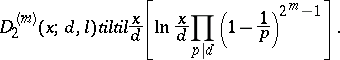In the general case it has been shown that :where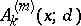is the expected principal term of growth,is a positive constant which may be arbitrary large, andis an arbitrary number.

The last inequality shows, in particular, that the sums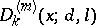, for any integers,, have, "on the average" , the same principal term of growth for all primitive arithmetical progressions with difference.